SUM AND DIFFERENCE IDENTITIES
DOUBLE ANGLE AND HALF ANGLE IDENTITIES

Example 1:

Show that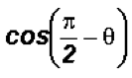can be changed to sin.

Let's use the Difference Identity for Cosine.

Here, u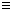and v.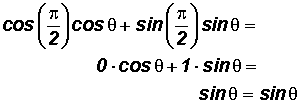We showed thatequals sin.

Example 2:

Show thatcan be changed to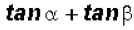.

Let's use the Sum Identity for Sine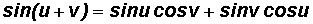.

Here, uand v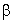.We showed thatequals.

Example 3:

Simplify.

Let's use the Sum and Difference Identities of Cosineand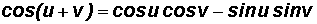.

Here, uand vx.We showed thatcan be simplified to.

Example 4:

Simplifyusing the Double Angle Identity.

Let's factor out a 3 to get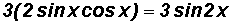.

We showed thatcan be written as 3 sin2x.

Example 5:

Simplify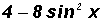using the Double Angle Identity.

Let's factor out a 4 to getWe showed thatcan be written as 4 cos2x.

Example 6:

Simplify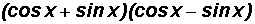using the Double Angle Identity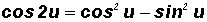.

Here, ux.

Let's multiply the parentheses using FOIL to get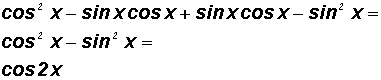We showed thatcan be written as cos 2x.

Example 7:

Simplify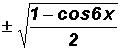using the Half Angle Identity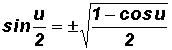.

We can see that u6x, therefore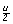3x.

Using the pattern of the Half Angle Identity, we can writeas sin 3x.

Example 8:

Simplify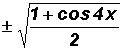using the Half Angle Identity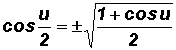.

We can see that u4x, therefore2x .

Using the pattern of the Half Angle Identity, we can writeas cos 2x.

Example 9:

Rewritein terms of a Double Angle Identity and a Half Angle Identity:

Using:

We can see that 2u6x, therefore u3x.

Using the pattern of the Double Angle Identity, we can writeas 2 sin 3x cos 3x.

Using:

We can see that6x, therefore u12x.

Using the pattern of the Half Angle Identity, we can writeas.